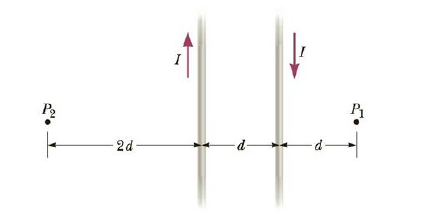Chapter 19, Problem 48P

Chapter
Section
Textbook Problem

The two wires shown in Figure P19.48 are separated by d = 10.0 cm and carry currents of I = 5.00 A in opposite directions. Find the magnitude and direction of the net magnetic field (a) at a point midway between the wires; (b) at point P1, 10.0 cm to the right of the wire on the right; and (c) at point P2, 2d = 20.0 cm to the left of the wire on the left.Figure P19.48

a)

To determine
The magnitude and direction of the net magnetic field at a point midway between the wires.

Explanation

Given info: The separation between the two wires is d=10.0cm . The current in the wires is I=5.00A and in opposite direction.

Explanation:

The arrangement of wires and points is shown in the figure.

The magnetic field due to a straight current carrying wire is given by,

B=μ0I2πr       (1)

• μ0 is the permeability of free space
• I is the current in the wire
• r is the distance of the point from the wire

Consider out of the page direction to be the positive direction.

At the midpoint of the two wires the magnetic field due to both the wire is directed into the page. The magnetic field due to both the wires is given by,

Bnet=B1B2

• B1 is the magnetic field due to the first wire
• B2 is the magnetic field due to the second wire

Using (1) the net magnetic field will be given by,

Bnet=μ0I2πr1μ0I2πr2

• r1 is the distance between the point and the first wire
• r2 is the distance between the point and the second wire

The distance between the two wires and the point is d2

b)

To determine
The magnitude and direction of the net magnetic field at a point P1 which is 10.0cm to the right of the wire on the right.

c)

To determine
The magnitude and direction of the net magnetic field at a point P2 which is 20.0cm to the left of the wire on the left.

Still sussing out bartleby?

Check out a sample textbook solution.

See a sample solution

The Solution to Your Study Problems

Bartleby provides explanations to thousands of textbook problems written by our experts, many with advanced degrees!

Get Started

What is the function of hCG?

Biology (MindTap Course List)

List the differences between mitosis and meiosis in the following chart:

Human Heredity: Principles and Issues (MindTap Course List)

What are the chemical differences between DNA and RNA?

Biology: The Dynamic Science (MindTap Course List)